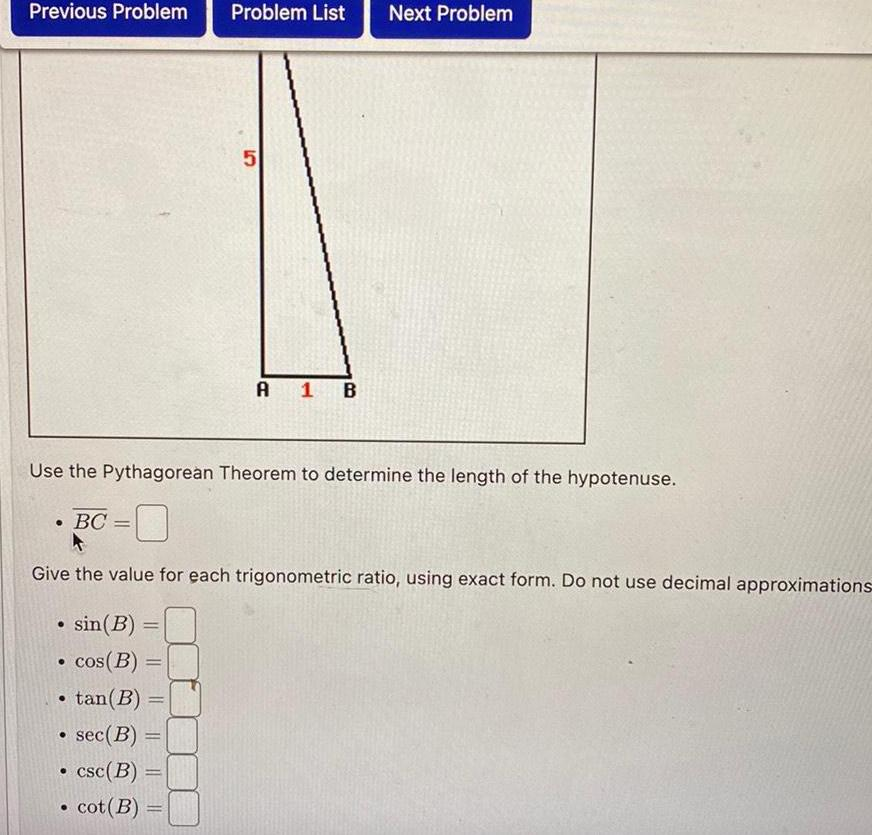Question:

# Previous Problem BC Use the Pythagorean Theorem to determine

Last updated: 11/19/2023Previous Problem BC Use the Pythagorean Theorem to determine the length of the hypotenuse Give the value for each trigonometric ratio using exact form Do not use decimal approximations sin B cos B tan B sec B csc B cot B Problem List 5 A 1 B Next Problem